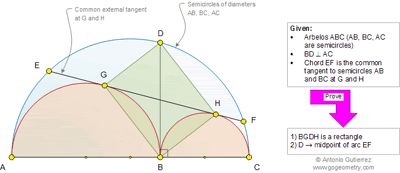## Wednesday, March 5, 2014

### Geometry Problem 989: Arbelos, Semicircles, Diameter, Perpendicular, 90 Degree, Common External Tangent, Rectangle, Midpoint of Arc

Geometry Problem. Post your solution in the comments box below.
Level: Mathematics Education, High School, Honors Geometry, College.

Click the figure below to view the complete problem 989#### 5 comments:

1.http://s25.postimg.org/vsrf9vrnj/Prob_989.png

Let M, L, N, O are points shown on the sketch
1. We have MG⊥GH and NH⊥GH
Since LG=LB=LH=> L is the midpoint of GH
∠(GBH)= ½(∠GMB+∠BNH)= 90
And ∠ (MLN)=1/2(∠GLB)+ ∠BLH)=90
In right triangle MLN , BL^2=BM.BN
In right triangle ADC , DB^2=BA.BC= 4. BM.BN=> L is the midpoint of BD
And DGBH is a parallelogram with right angle ∠GBH => DGBH is a rectangle
2. Connect AG and CH
We have ∠ (AGB=∠ (BGD)= 90 => A,G,D are collinear
Triangles AMG and AOD are isosceles with common angle ∠GAM
So ∠ (AGM)= ∠ (ADO) => OD//MG => OD ⊥ EF
So D is the midpoint of arc EF

2.I have a comment on the second part.
DO || HN, and they are perpendicular to EF.
So DO bisects both chord EF and arc EF.

1.Thank you for your comment on my solution.
Peter

3.Because <GAC=<FHC, AGHC is cyclic. Let AG and HC meet at D'. <D'AC+<D'CA=90, so D' must be on big circle. Furthermore, because D'G*D'A=D'H*D'C from cyclic property of AGHC, D' must also be on radical axis of 2 small circles, which is their common tangent. Therefore, D' coincides with D. It is easy to see that GDHB has 3 right angles from Thales theorem in each circle and must therefore be rectangle.
<GAC=<FHC=arcDF/2+arcFC/2=arcDE+arcFC/2.
This means that arcDF=arcDE so D is midpoint of arcEF.

4.Draw a circle with diameter BD. It meets (AB) in X and (BC) in Y.

<BXD=<BYD=90 deg by Thales' theorem.
<BXA=<BYC=90 deg by Thales' theorem.
So X lies on AD and Y lies on BD.
Thales again: <XDY = <ADC = 90 deg.
Now note that <BXY = 90 deg - <BDX = <BAX.
So XY is tangent to the (AB) at X and similarly tangent to (BC) at Y. So X=G and Y=H.

By similarity the tangent to the (AC) at D is parallel to GH. Let M be the midpoint of {AC), then MD is perpendicular to EF, hence bisects EF and bisects angle EMF, and D is midpoint of arc EF.